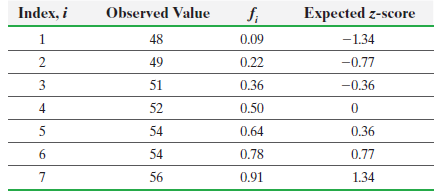×
Get Full Access to Statistics: Informed Decisions Using Data - 5 Edition - Chapter 7 - Problem 14
Get Full Access to Statistics: Informed Decisions Using Data - 5 Edition - Chapter 7 - Problem 14

×

# ?Use the results in the table to (a) draw a normal plot, (b) determine the linear correlation between the observed values and expected z-scores, (c) deISBN: 9780134133539 240

## Solution for problem 14 Chapter 7

Statistics: Informed Decisions Using Data | 5th Edition

• Textbook Solutions
• 2901 Step-by-step solutions solved by professors and subject experts
• Get 24/7 help from StudySoup virtual teaching assistantsStatistics: Informed Decisions Using Data | 5th Edition

4 5 1 365 Reviews
20
4
Problem 14

Use the results in the table to (a) draw a normal plot, (b) determine the linear correlation between the observed values and expected z-scores, (c) determine the critical value in Table VI to assess the normality of the data.Step-by-Step Solution:

Step 1 of 5) Use the results in the table to (a) draw a normal plot, (b) determine the linear correlation between the observed values and expected z-scores, (c) determine the critical value in Table VI to assess the normality of the data. Notice that Excel provides a correlation matrix, which means that for every pair of columns in the spreadsheet it will compute and display the correlation in the bottom triangle of the matrix. Because the linear correlation coefficient is positive, we know above-average values of x, club-head speed, are associated with above-average values of y, driving distance, and below-average values of x are associated with below-average values of y.

Step 2 of 2

##### ISBN: 9780134133539

The answer to “?Use the results in the table to (a) draw a normal plot, (b) determine the linear correlation between the observed values and expected z-scores, (c) determine the critical value in Table VI to assess the normality of the data.” is broken down into a number of easy to follow steps, and 39 words. This full solution covers the following key subjects: . This expansive textbook survival guide covers 88 chapters, and 2422 solutions. Since the solution to 14 from 7 chapter was answered, more than 216 students have viewed the full step-by-step answer. Statistics: Informed Decisions Using Data was written by and is associated to the ISBN: 9780134133539. The full step-by-step solution to problem: 14 from chapter: 7 was answered by , our top Statistics solution expert on 01/15/18, 03:19PM. This textbook survival guide was created for the textbook: Statistics: Informed Decisions Using Data, edition: 5.

## Discover and learn what students are asking

Statistics: Informed Decisions Using Data : The Design of Experiments
?What is replication in an experiment?

Statistics: Informed Decisions Using Data : Testing the Significance of the Least-Squares Regression Model
?Tar and Nicotine Every year the Federal Trade Commission (FTC) must report tar and nicotine levels in cigarettes to Congress. Tar and nicotine levels

Unlock Textbook Solution